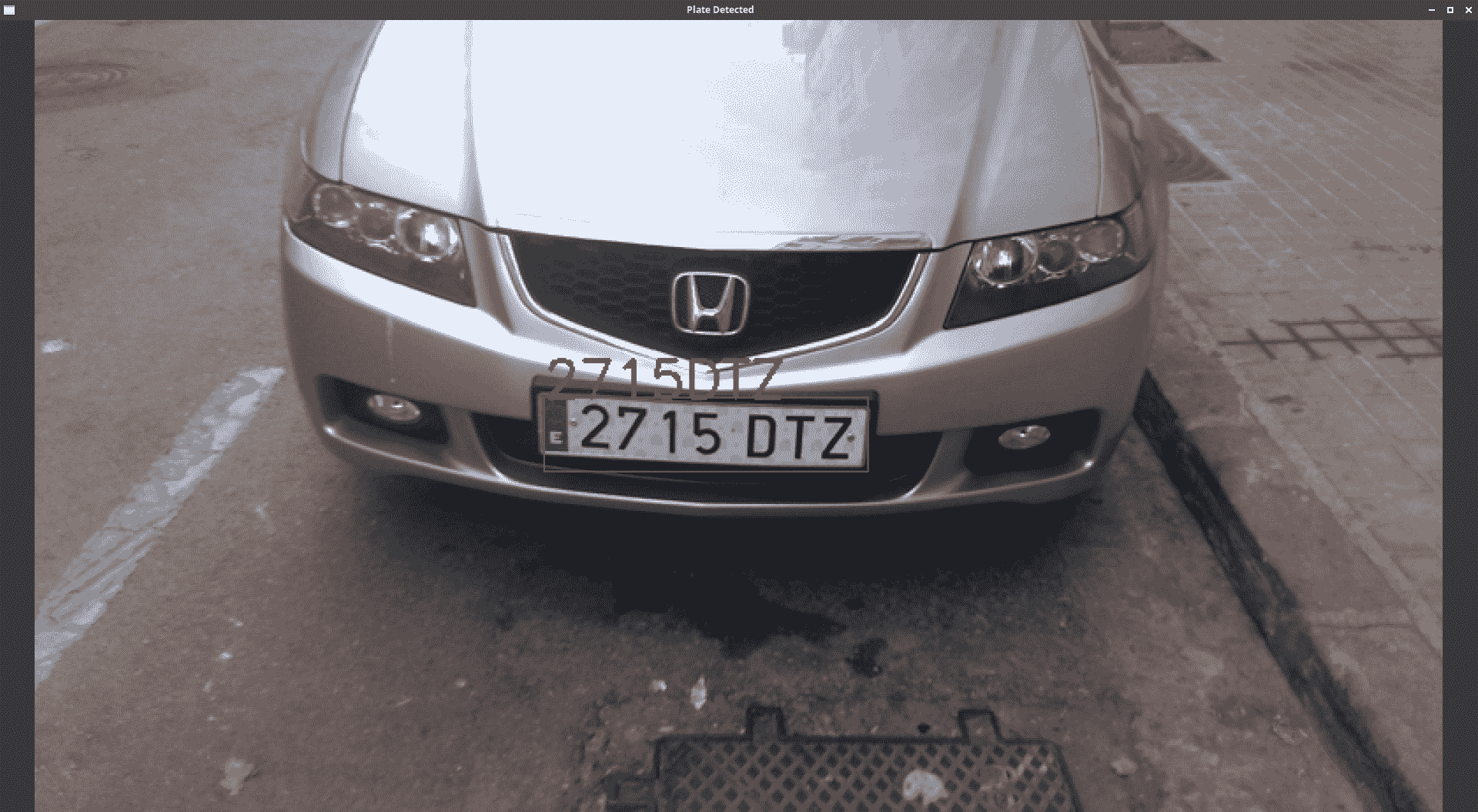• ANPR
• 板材检测
• 车牌识别

# ANPR 简介

ANPR 有时也被称为自动车牌识别、(ALPR)、自动车辆识别、(AVI)或车牌识别、(CPR)，是一种智能监控方法，它使用光学字符识别和(OCR)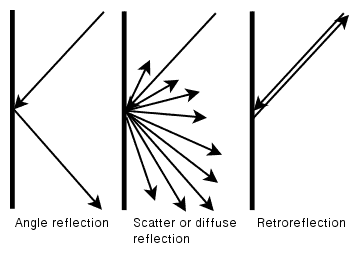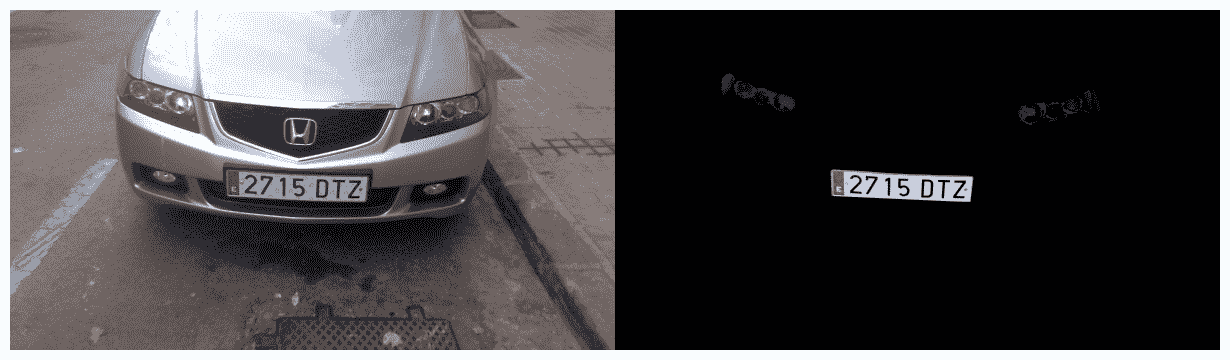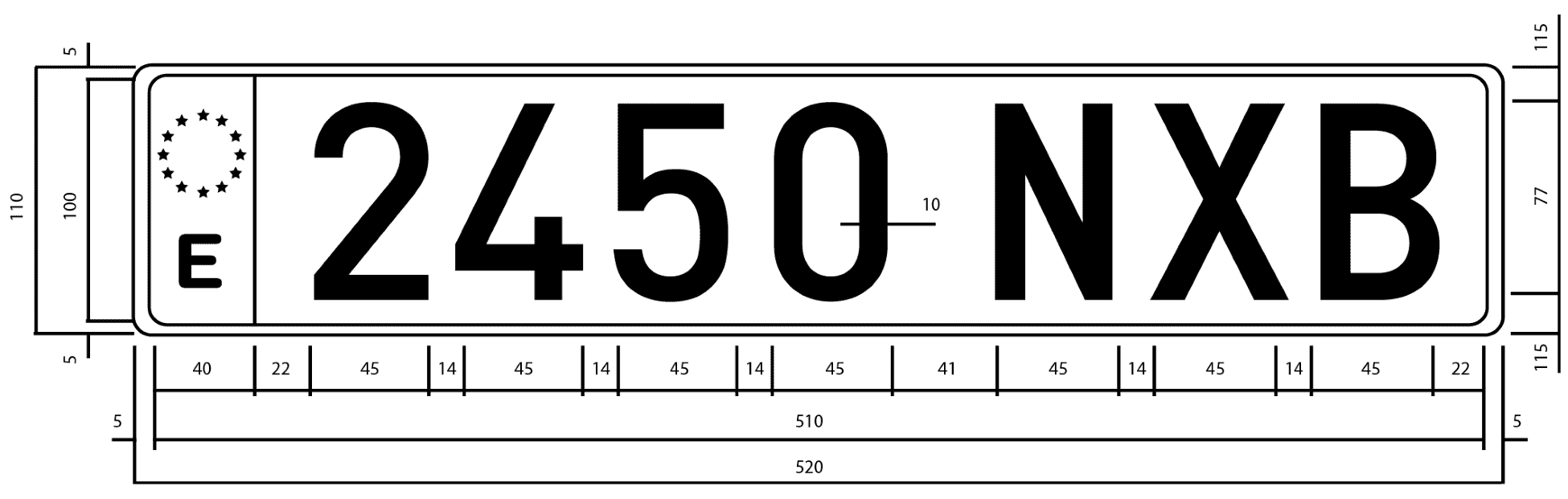# ANPR 算法

• 车牌检测的目的是检测车牌在整个摄像机画面中的位置。
• 当在图像中检测到车牌时，车牌分段被传递到第二步(车牌识别)，该步骤使用 OCR 算法来确定车牌上的字母数字字符。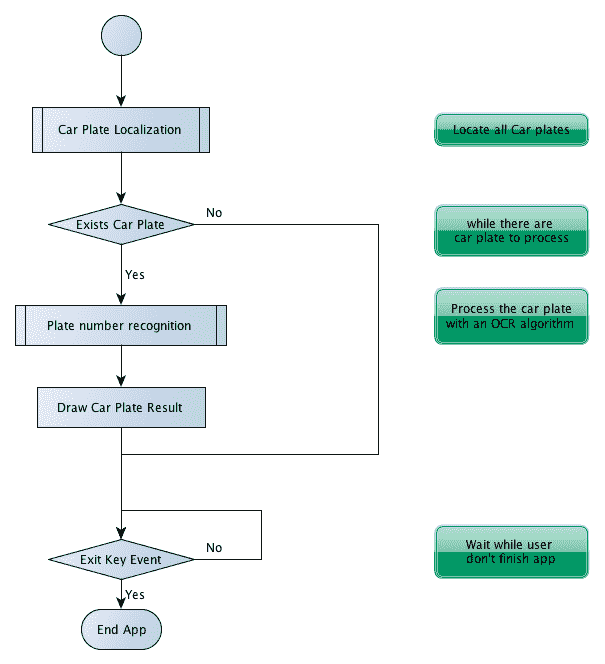1. 分割：此步骤检测并移除图像中的每个感兴趣的补丁/区域。
2. 特征提取：此步骤从每个补丁中提取一组特征。
3. 分类：该步骤从车牌识别步骤中提取每个字符，或者在车牌检测步骤中将每个图像块分类为车牌无车牌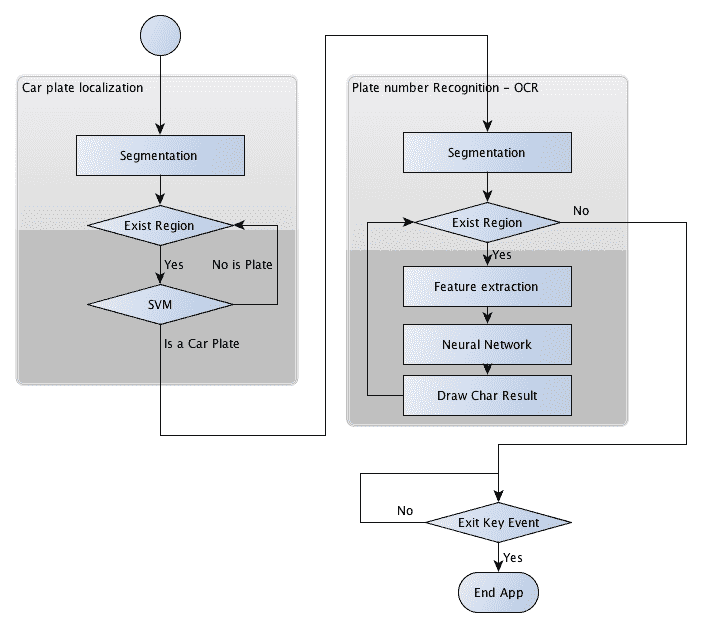• 如何训练模式识别系统
• 如何评价它？

# 板材检测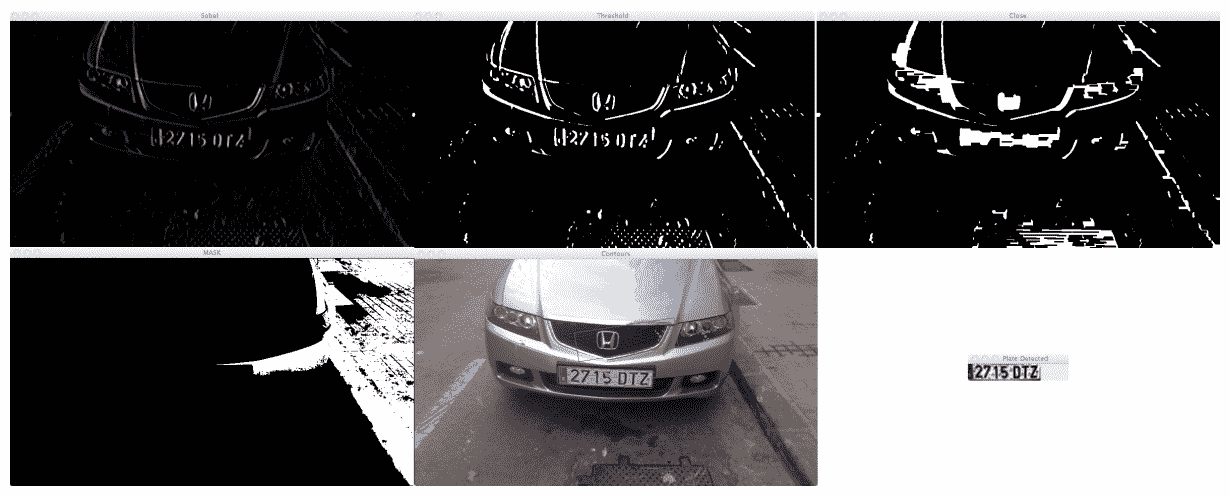• Sobel 滤波器
• 阈值操作
• 封闭形态运算
• 其中一个填充区域的蒙版
• 红色，可能检测到的车牌(图片特色)
• 用支持向量机分类器检测车牌

# 分割

//convert image to gray
Mat img_gray;
cvtColor(input, img_gray, CV_BGR2GRAY);
blur(img_gray, img_gray, Size(5,5));


void Sobel(InputArray src, OutputArray dst, int ddepth, int xorder, int yorder, int ksize=3, double scale=1, double delta=0, int borderType=BORDER_DEFAULT )


//Find vertical lines. Car plates have high density of vertical
lines
Mat img_sobel;
Sobel(img_gray, img_sobel, CV_8U, 1, 0, 3, 1, 0);


//threshold image
Mat img_threshold;
threshold(img_sobel, img_threshold, 0, 255, CV_THRESH_OTSU+CV_THRESH_BINARY);


When the CV_THRESH_OTSU value is defined, the threshold function returns the optimal threshold value obtained by Otsu's algorithm.

Mat element = getStructuringElement(MORPH_RECT, Size(17, 3));


morphologyEx(img_threshold, img_threshold, CV_MOP_CLOSE, element);


//Find contours of possibles plates
vector< vector< Point>> contours;
findContours(img_threshold,
contours, // a vector of contours
CV_RETR_EXTERNAL, // retrieve the external contours
CV_CHAIN_APPROX_NONE); // all pixels of each contours


//Start to iterate to each contour founded
vector<vector<Point>>::iterator itc= contours.begin();
vector<RotatedRect> rects;

//Remove patch that has no inside limits of aspect ratio and
area.
while (itc!=contours.end()) {
//Create bounding rect of object
RotatedRect mr= minAreaRect(Mat(*itc));
if(!verifySizes(mr)){
itc= contours.erase(itc);
}else{
++ itc;
rects.push_back(mr);
}
}


bool DetectRegions::verifySizes(RotatedRect candidate ){
float error=0.4;
//Spain car plate size: 52x11 aspect 4,7272
const float aspect=4.7272;
//Set a min and max area. All other patchs are discarded
int min= 15*aspect*15; // minimum area
int max= 125*aspect*125; // maximum area
//Get only patches that match to a respect ratio.
float rmin= aspect-aspect*error;
float rmax= aspect+aspect*error;

int area= candidate.size.height * candidate.size.width;
float r= (float)candidate.size.width
/(float)candidate.size.height;
if(r<1)
r= 1/r;

if(( area < min || area > max ) || ( r < rmin || r > rmax )){
return false;
}else{
return true;
}
}


for(int i=0; i< rects.size(); i++){
//For better rect cropping for each possible box
//Make floodFill algorithm because the plate has white background
//And then we can retrieve more clearly the contour box
circle(result, rects[i].center, 3, Scalar(0,255,0), -1);
//get the min size between width and height
float minSize=(rects[i].size.width < rects[i].size.height)?
rects[i].size.width:rects[i].size.height;
minSize=minSize-minSize*0.5;
//initialize rand and get 5 points around center for floodFill algorithm
srand ( time(NULL) );
//Initialize floodFill parameters and variables
mask.create(input.rows + 2, input.cols + 2, CV_8UC1);
int loDiff = 30;
int upDiff = 30;
int connectivity = 4;
int NumSeeds = 10;
Rect ccomp;
int flags = connectivity + (newMaskVal << 8 ) + CV_FLOODFILL_FIXED_RANGE + CV_FLOODFILL_MASK_ONLY;
for(int j=0; j<NumSeeds; j++){
Point seed;
seed.x=rects[i].center.x+rand()%(int)minSize-(minSize/2);
seed.y=rects[i].center.y+rand()%(int)minSize-(minSize/2);
circle(result, seed, 1, Scalar(0,255,255), -1);
int area = floodFill(input, mask, seed, Scalar(255,0,0), &ccomp, Scalar(loDiff, loDiff, loDiff), Scalar(upDiff, upDiff, upDiff), flags);


int floodFill(InputOutputArray image, InputOutputArray mask, Point seed, Scalar newVal, Rect* rect=0, Scalar loDiff=Scalar(), Scalar upDiff=Scalar(), int flags=4 )


• 低位：这些位包含函数中使用的连接值，四个(默认情况下)或八个。 连通性确定要考虑像素的哪些邻居。
• 高位：这些位可以是 0，也可以是下列值的组合：CV_FLOODFILL_FIXED_RANGE和*CV_FLOODFILL_MASK_ONLY

CV_FLOODFILL_FIXED_RANGE设置当前像素和种子像素之间的差异。CV_FLOODFILL_MASK_ONLY将只填充图像蒙版，不会更改图像本身。

//Check new floodFill mask match for a correct patch.
//Get all points detected for get Minimal rotated Rect
vector<Point> pointsInterest;
RotatedRect minRect = minAreaRect(pointsInterest);
if(verifySizes(minRect)){


//Get rotation matrix
float r= (float)minRect.size.width / (float)minRect.size.height;
float angle=minRect.angle;
if(r<1)
angle=90+angle;
Mat rotmat= getRotationMatrix2D(minRect.center, angle,1);


//Create and rotate image
Mat img_rotated;
warpAffine(input, img_rotated, rotmat, input.size(),
CV_INTER_CUBIC);


//Crop image
Size rect_size=minRect.size;
if(r < 1)
swap(rect_size.width, rect_size.height);
Mat img_crop;
getRectSubPix(img_rotated, rect_size, minRect.center, img_crop);


Mat resultResized;
resultResized.create(33,144, CV_8UC3);
resize(img_crop, resultResized, resultResized.size(), 0, 0, INTER_CUBIC);
//Equalize croped image
Mat grayResult;
cvtColor(resultResized, grayResult, CV_BGR2GRAY);
blur(grayResult, grayResult, Size(3,3));
equalizeHist(grayResult, grayResult);


output.push_back(Plate(grayResult,minRect.boundingRect()));


# 分类 / 同 taxonomy / 种类 / 类别，等级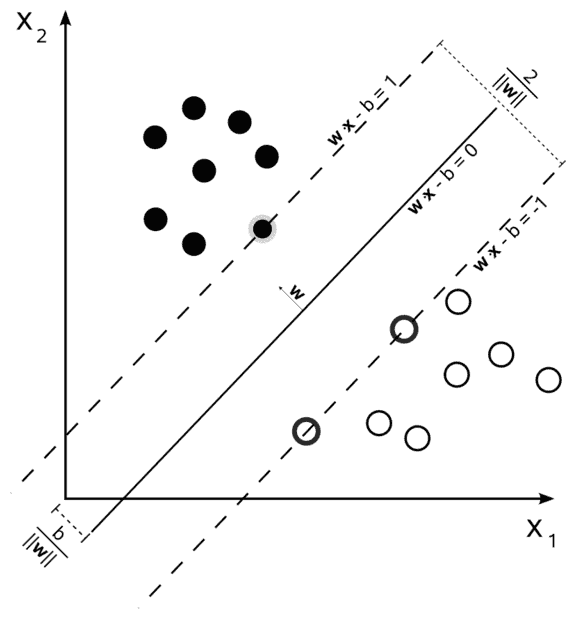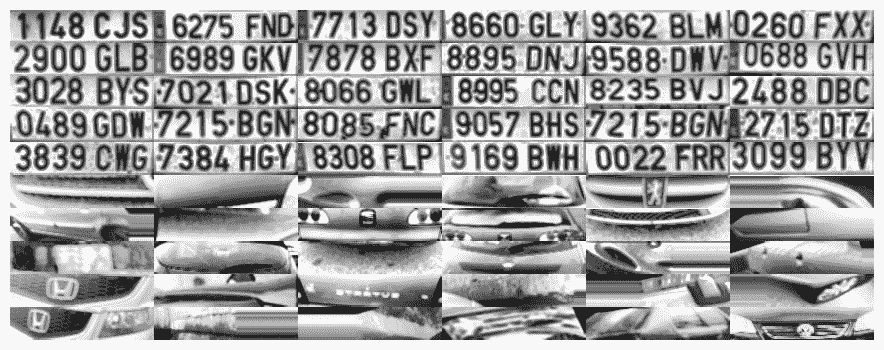Training data for a machine learning OpenCV algorithm is stored in an NxM matrix, with N samples and M features. Each dataset is saved as a row in the training matrix.

OpenCV 提供了一种非常简单的方法来管理 XML 或 YAML 格式的数据文件，它使用FileStorage类。 这个类允许我们存储和读取 OpenCV 变量和结构，或者我们的自定义变量。 使用此功能，我们可以读取培训数据矩阵和培训课程，并将其保存在SVM_TrainingDataSVM_Classes中：

FileStorage fs;
Mat SVM_TrainingData;
Mat SVM_Classes;
fs["TrainingData"] >>SVM_TrainingData;
fs["classes"] >>SVM_Classes;


Ptr<TrainData> trainData = TrainData::create(SVM_TrainingData, ROW_SAMPLE, SVM_Classes);


Ptr<SVM> svmClassifier = SVM::create()


svmClassifier->setTermCriteria(TermCriteria(TermCriteria::MAX_ITER, 1000, 0.01));
svmClassifier->setC(0.1);
svmClassifier->setKernel(SVM::LINEAR);


svmClassifier->train(trainData);


vector<Plate> plates;
for(int i=0; i< posible_regions.size(); i++)
{
Mat img=posible_regions[i].plateImg;
Mat p= img.reshape(1, 1);//convert img to 1 row m features
p.convertTo(p, CV_32FC1);
int response = (int)svmClassifier.predict( p );
if(response==1)
plates.push_back(posible_regions[i]);
}


# OCR 分割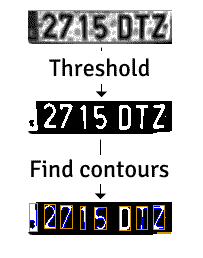Mat img_threshold;
threshold(input, img_threshold, 60, 255, CV_THRESH_BINARY_INV);
if(DEBUG)
imshow("Threshold plate", img_threshold);
Mat img_contours;
img_threshold.copyTo(img_contours);
//Find contours of possibles characters
vector< vector< Point>> contours;
findContours(img_contours, contours, // a vector of contours
CV_RETR_EXTERNAL, // retrieve the external contours
CV_CHAIN_APPROX_NONE); // all pixels of each contours


bool OCR::verifySizes(Mat r){
//Char sizes 45x77
float aspect=45.0f/77.0f;
float charAspect= (float)r.cols/(float)r.rows;
float error=0.35;
float minHeight=15;
float maxHeight=28;
//We have a different aspect ratio for number 1, and it can be ~0.2
float minAspect=0.2;
float maxAspect=aspect+aspect*error;
//area of pixels
float area=countNonZero(r);
//bb area
float bbArea=r.cols*r.rows;
//% of pixel in area
float percPixels=area/bbArea;
if(percPixels < 0.8 && charAspect > minAspect && charAspect <
maxAspect && r.rows >= minHeight && r.rows < maxHeight)
return true;
else
return false;
}


# 基于卷积神经网络的字符分类

• 卷积：本层对输入应用卷积运算过滤器，将结果传递给下一层。 这一层的工作原理类似于典型的计算机视觉过滤器(Sobel、Canny 等)，但内核过滤器是在训练阶段学习的。 使用这一层的主要好处是减少了常见的完全连接的前馈神经网络，例如，100 x 100 图像有 10,000 个权重，但使用 CNN，问题就减少到了核大小；例如，应用 5 x 5 和 32 个不同过滤器的核，只有5532=800。 同时，这些过滤器激发了特征提取的所有可能性。
• 汇集：这一层将一组神经元的输出合并成一个单一的。 最常见的是 max pooling，它返回输入神经元组的最大值。 深度学习中另一种经常使用的方法是平均汇集。 这一层为 CNN 带来了在随后的层中提取更高级别特征的可能性。
• FLATEN：FLATEN 不是 DNN 层，而是将矩阵转换为简单向量的常见操作；此步骤是应用其他层并最终获得分类所必需的。
• 完全连接：这与传统的多层感知器相同，在传统的多层感知器中，前一层的每个神经元都通过激活功能连接到下一层。
• Dropout：-这一层是减少过拟合的正则化；-它是对模型执行精确度的常用层。
• 损失层：这通常是 DNN 中的最后一层，并指定如何训练和计算误差以执行预测。 一种非常常见的损失层是用于分类的 Softmax。

OpenCV 深度学习并不是为培训深度学习模型而设计的，也不受支持，因为有非常稳定和强大的开源项目只专注于深度学习，如 TensorFlow、Caffe 和 Torch。 然后，OpenCV 有一个接口来导入和读取最重要的模型。

# 准备数据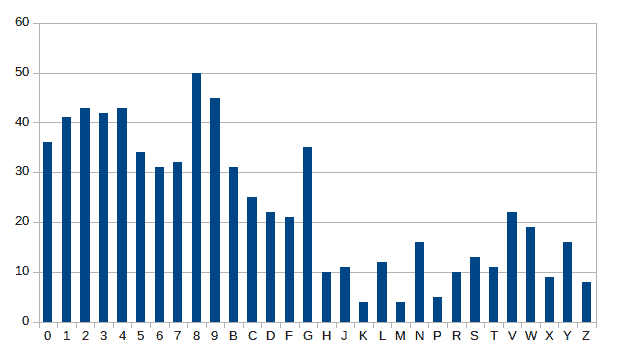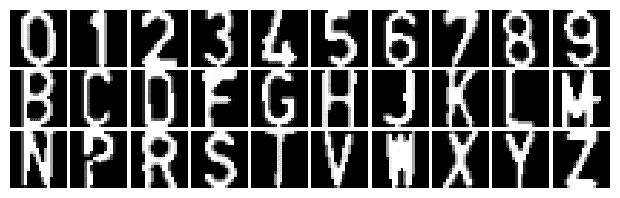pip install Augmentor


import Augmentor
number_samples = 20000
p = Augmentor.Pipeline("./chars_seg/chars/")
p.random_distortion(probability=0.4, grid_width=4, grid_height=4, magnitude=1)
p.shear(probability=0.5, max_shear_left=5, max_shear_right=5)
p.skew_tilt(probability=0.8, magnitude=0.1)
p.rotate(probability=0.7, max_left_rotation=5, max_right_rotation=5)
p.sample(number_samples)


pip install tensorflow


pip install tensorflow-gpu


python ./create_tfrecords_from_dir.py -i ../data/chars_seg/DNN_data/test -o ../data/chars_seg/DNN_data/test.tfrecords
python ./create_tfrecords_from_dir.py -i ../data/chars_seg/DNN_data/train -o ../data/chars_seg/DNN_data/train.tfrecords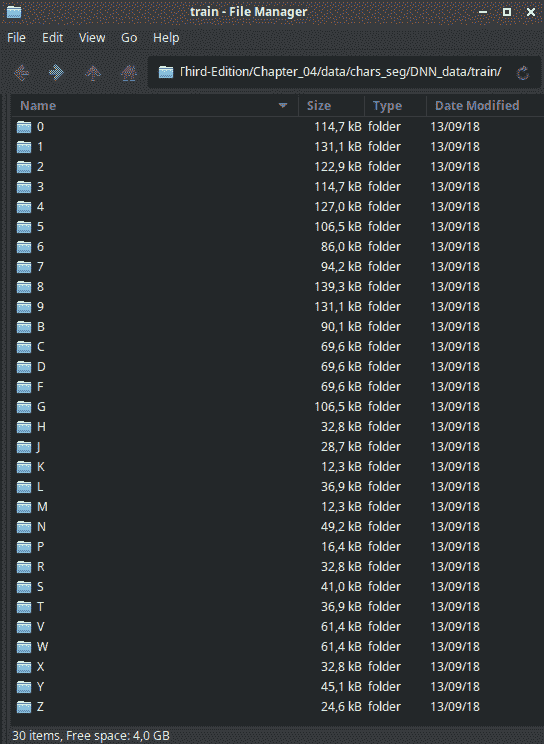# 创建 TensorFlow 模型

TensorFlow 是一个开源软件库，专注于高性能数值计算和深度学习，可访问和支持 CPU、GPU 和 TPU(张量处理单元，专用于深度学习的新 Google 硬件)。 这个库不是一个容易的库，学习曲线很高，但引入 Kera(TensorFlow 之上的库)作为 TensorFlow 的一部分，让学习曲线变得更容易，但仍然需要巨大的学习曲线本身。

• 卷积层 1：32 个 5x5 带 REU 激活功能的滤波器
• 池化第 2 层：使用 2 x 2 过滤器和跨度为 2 的最大池化
• 卷积层 3：64 个 5x5 滤波器，具有 RELU 激活功能
• 池化层 4：使用 2 x 2 过滤器和跨度为 2 的最大池化
• 致密层 5：↔1,024 个神经元
• 丢包层 6：丢包率为 0.4 的丢包率正则化
• 致密层 7：包含30 个神经元，每个数字和字符对应一个神经元
• SoftMax Layer 8：SoftMax Layer Lost 函数，采用梯度下降优化器，学习率为 0.001，训练步数为 20,000 步。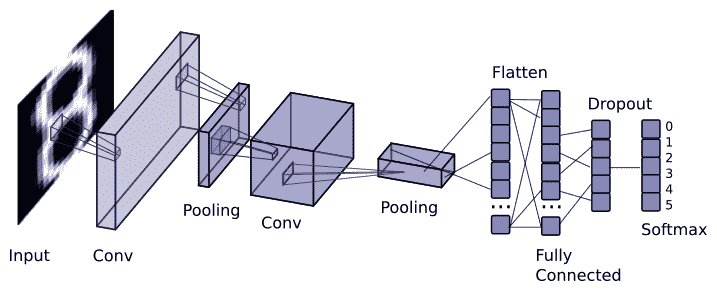TensorEditor 是一个在线工具，它允许我们为 TensorFlow 创建模型并在云上进行训练，或者下载 Python2.7 代码并在本地执行。 注册在线免费下载工具后，即可生成模型，如下图所示：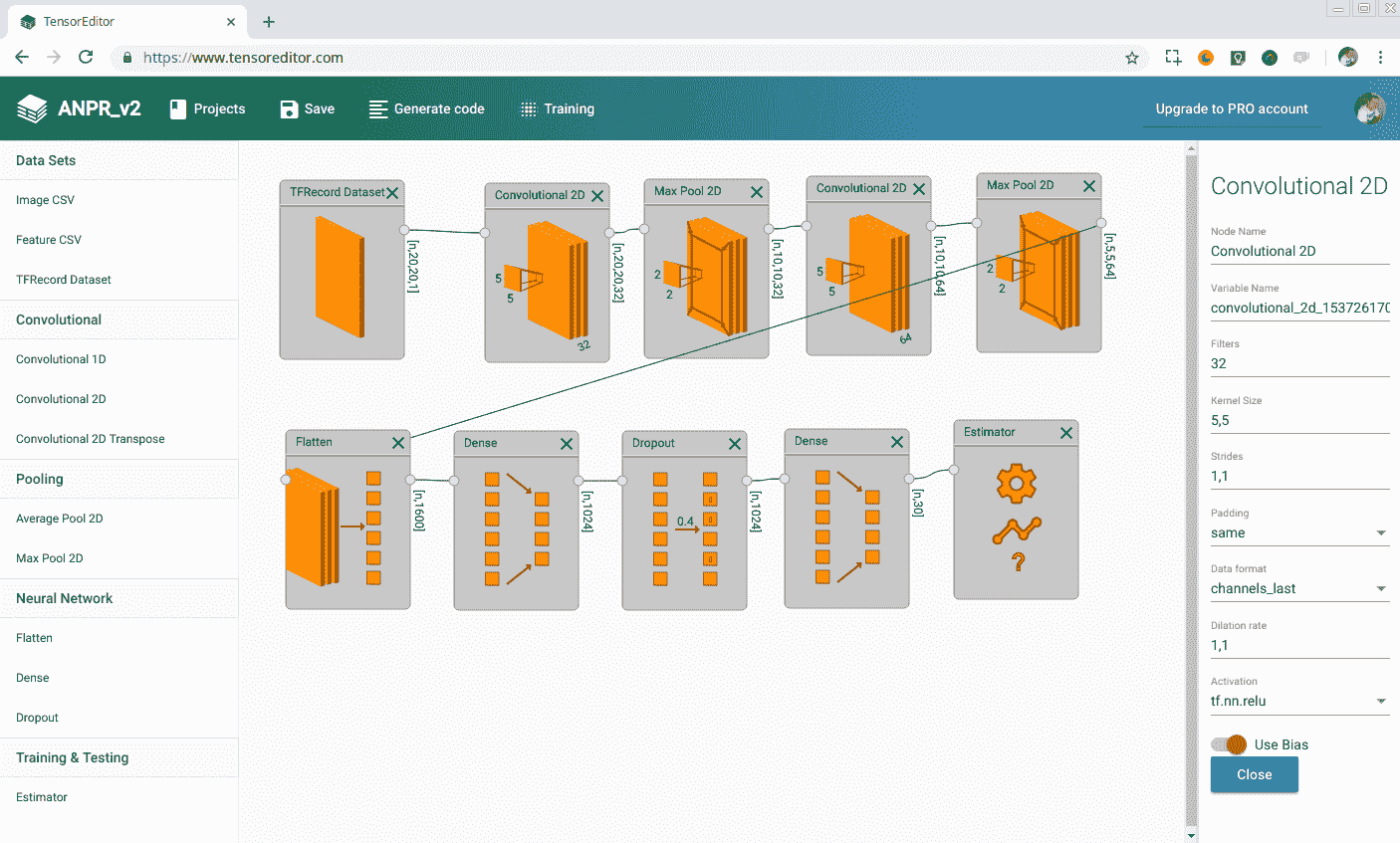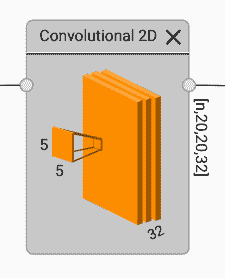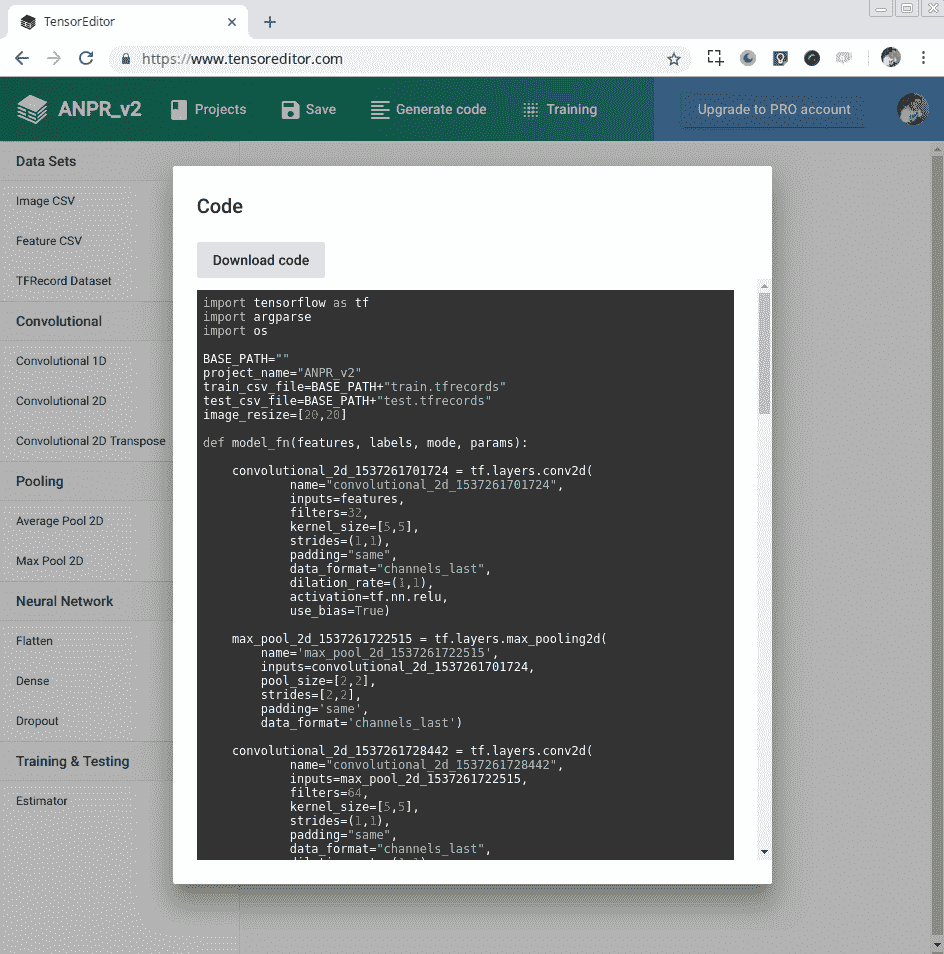python code.py --job-dir=./model_output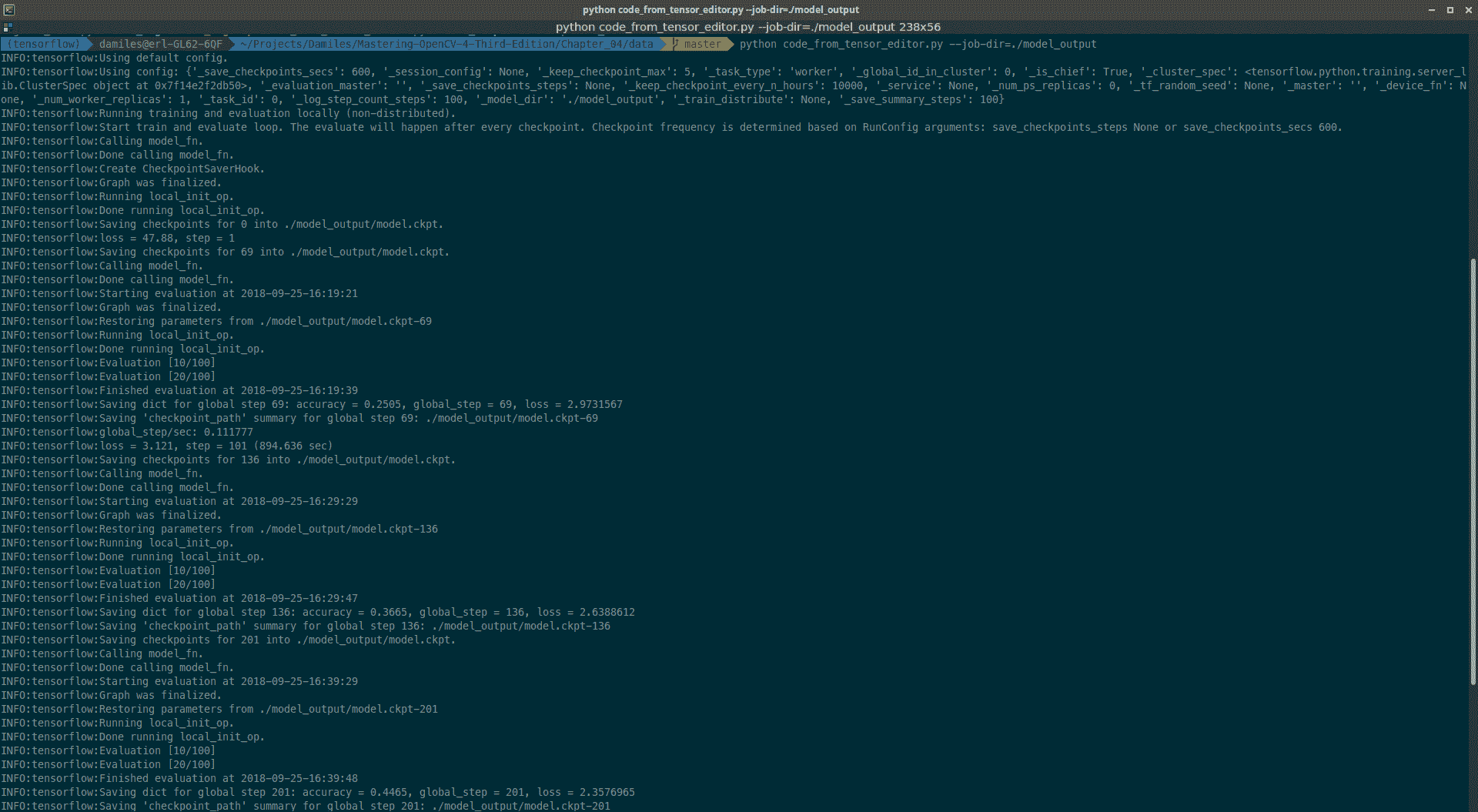Output of the algorithm training command

tensorboard --logdir ./model_output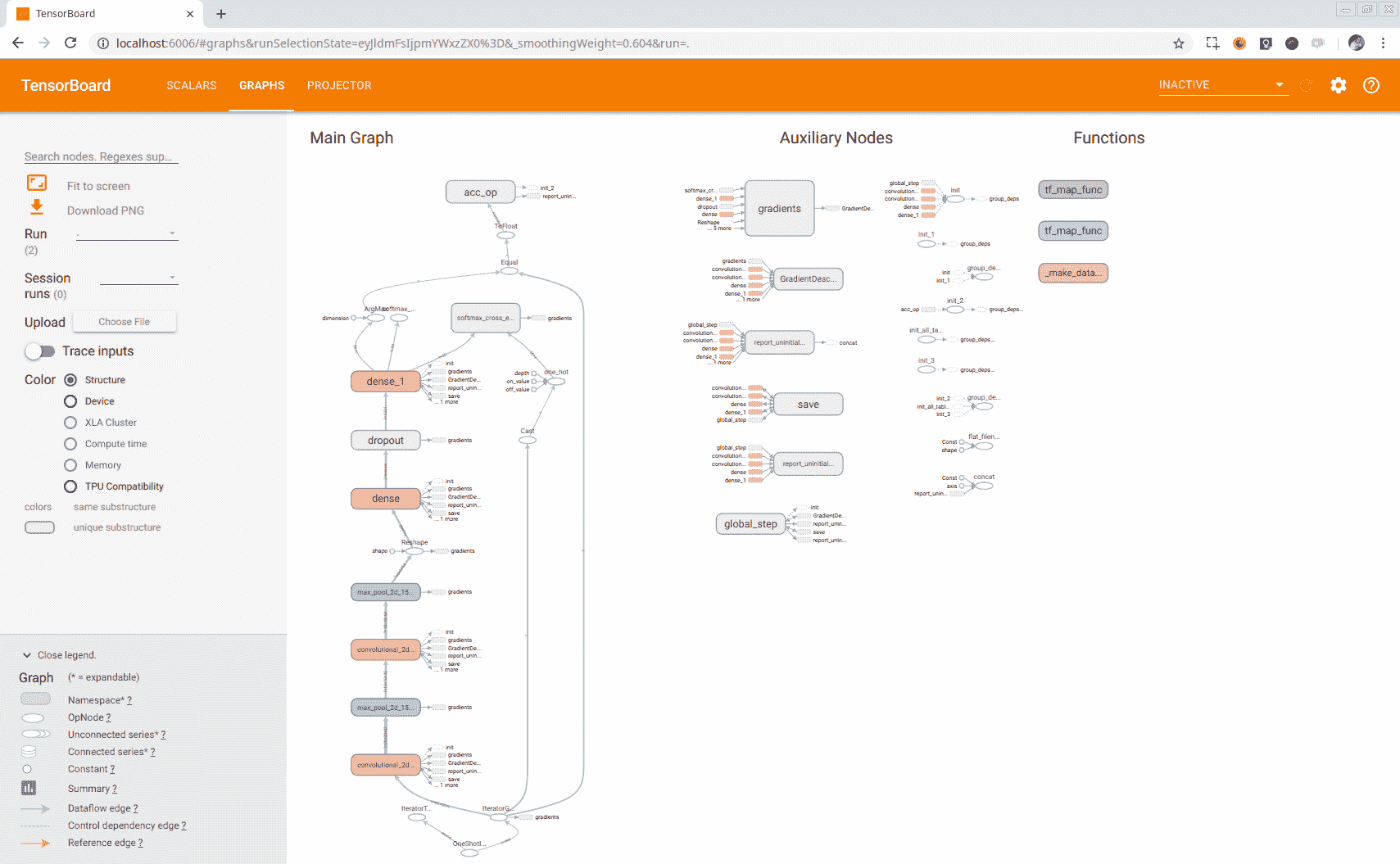TensorBoard GRAPHS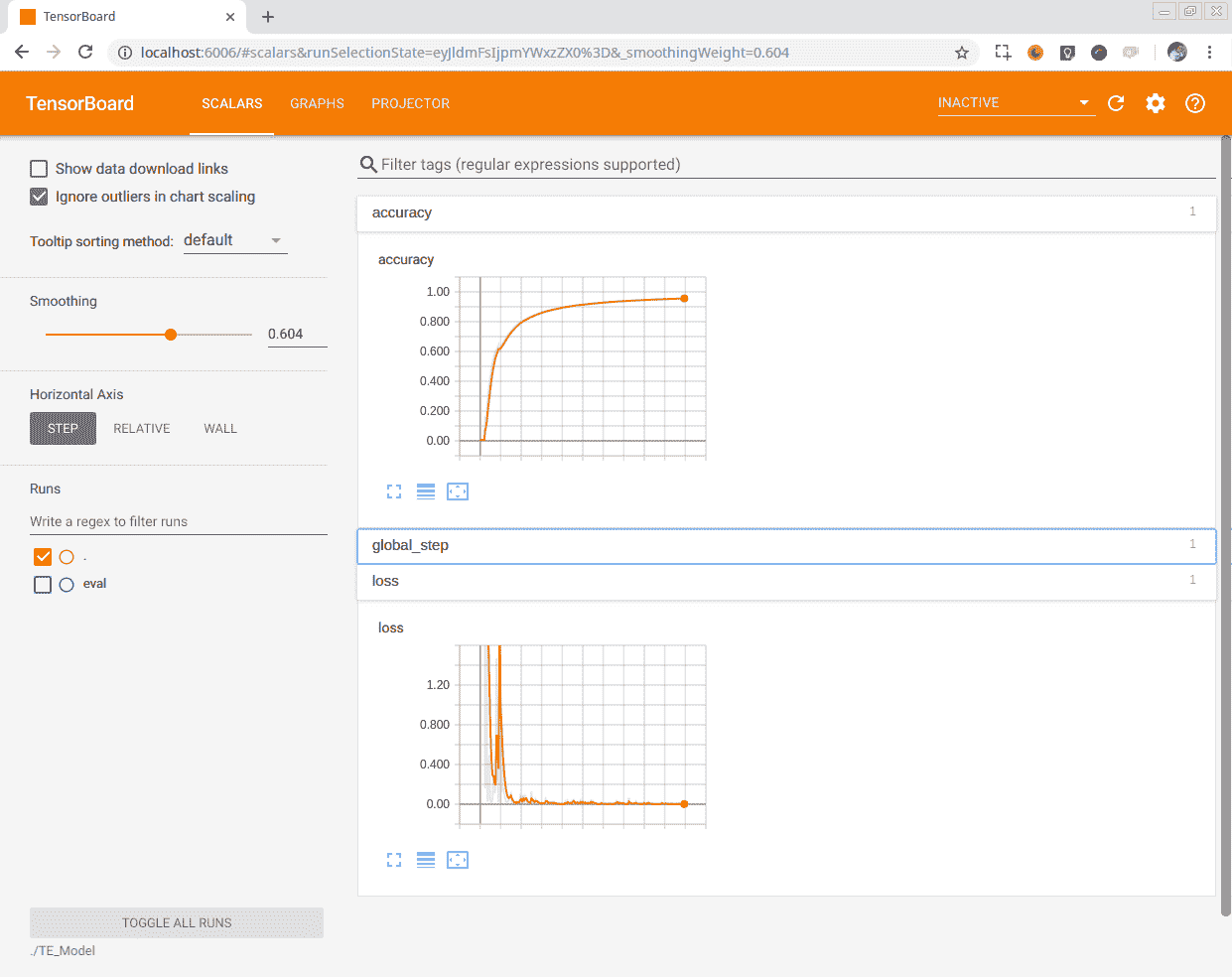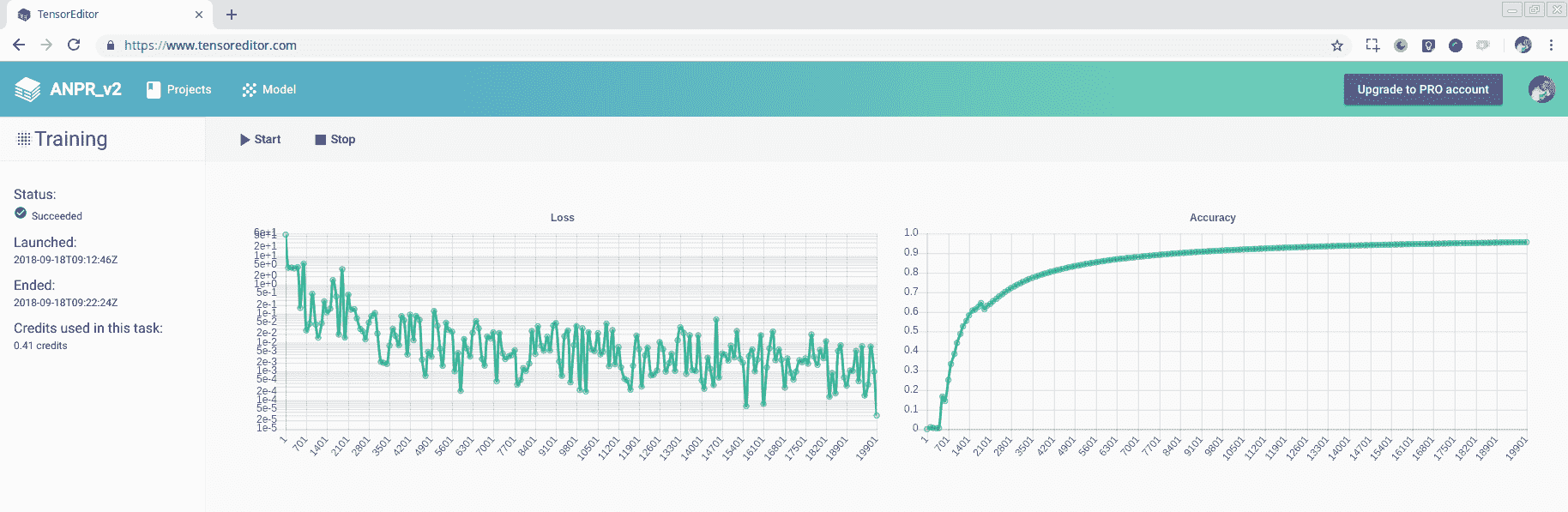Training in TensorEditor

# 为 OpenCV 准备模型

TensorFlow 在我们训练新模型时会生成多个文件，为存储每个步骤中获得的准确性和损失以及其他指标的事件生成文件；此外，一些文件还会存储每个步骤或检查点获得的变量结果。 这些变量是网络在训练中学习的权重。 但在生产中共享所有这些文件并不方便，因为 OpenCV 无法管理它们。 同时，还有一些节点只用于训练而不用于推理。 我们必须从模型中删除这些节点，例如 Dropout 层或训练输入迭代器。

• 冻结我们的图表
• 移除不需要的节点/层
• 针对推理进行优化

freeze_graph --input_graph=graph.pbtxt --input_checkpoint=model.ckpt-20000 --output_graph frozen_graph.pb --output_node_names=softmax_tensor


transform_graph --in_graph="frozen_graph.pb" --out_graph="frozen_cut_graph.pb" --inputs="IteratorGetNext" --outputs="softmax_tensor" --transforms='strip_unused_nodes(type=half, shape="1,20,20,1") fold_constants(ignore_errors=true) fold_batch_norms fold_old_batch_norms sort_by_execution_order'


It's very important to add the sort_by_execution_order parameter to ensure that the layers are stored in order in the model graph, to allow OpenCV to correctly import the model. OpenCV sequentially imports the layers from the graph model, checking that all previous layers, operations, or variables are imported; if not, we will receive an import error. TensorEditor doesn't take care of the execution order in the graph to construct and execute it.

optimize_for_inference.py --input frozen_cut_graph.pb --output frozen_cut_graph_opt.pb --frozen_graph True --input_names IteratorGetNext --output_names softmax_tensor


# 在 OpenCV C++ 代码中导入和使用模型

dnn::Net dnn_net= readNetFromTensorflow("frozen_cut_graph_opt.pb");


for(auto& segment : segments){
//Preprocess each char for all images have same sizes
Mat ch=preprocessChar(segment.img);
// DNN classify
Mat inputBlob;
blobFromImage(ch, inputBlob, 1.0f, Size(20, 20), Scalar(), true, false);
dnn_net.setInput(inputBlob);

Mat outs;
dnn_net.forward(outs);
cout << outs << endl;
double max;
Point pos;
minMaxLoc( outs, NULL, &max, NULL, &pos);
cout << "---->" << pos << " prob: " << max << " " << strCharacters[pos.x] << endl;

input->chars.push_back(strCharacters[pos.x]);
input->charsPos.push_back(segment.pos);
}



void cv::dnn::blobFromImage (
InputArray image,
OutputArray blob,
double scalefactor = 1.0,
const Size & size = Size(),
const Scalar & mean = Scalar(),
bool swapRB = false,
bool crop = false,
int ddepth = CV_32F
)


• image：输入图像(具有一个、三个或四个通道)。
• blob：输出斑点垫。
• size：输出图像的空间大小。
• mean：标量和平均值，从通道中减去。 如果图像具有 BGR 排序，并且swapRBtrue，则值应该是(Mean-R，Mean-G，Mean-B)顺序。
• scalefactor：图像值的乘数。
• swapRB：指示需要交换三通道图像中的第一个通道和最后一个通道的标志。
• crop：指示调整大小后是否裁剪图像的标志
• ddepth：输出深度blob。 选择CV_32FCV_8U